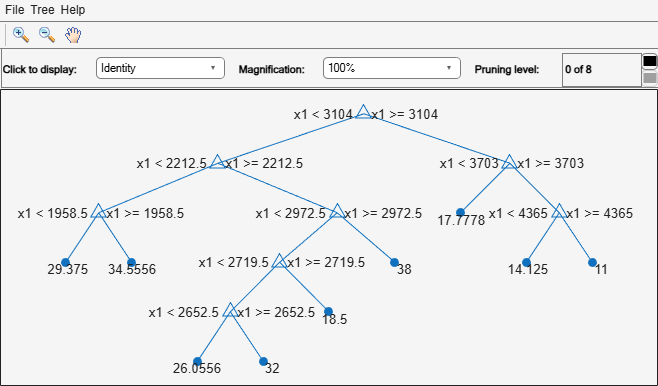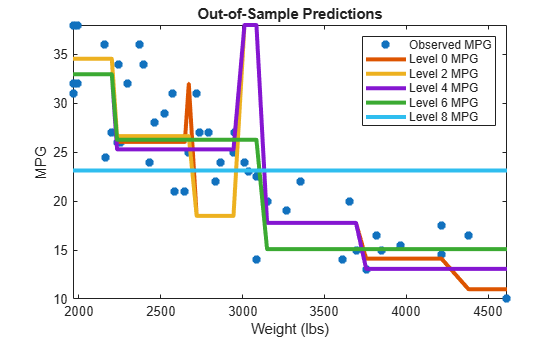# Predict Out-of-Sample Responses of Subtrees

This example hows how to predict out-of-sample responses of regression trees, and then plot the results.

Load the `carsmall` data set. Consider `Weight` as a predictor of the response `MPG`.

```load carsmall idxNaN = isnan(MPG + Weight); X = Weight(~idxNaN); Y = MPG(~idxNaN); n = numel(X);```

Partition the data into training (50%) and validation (50%) sets.

```rng(1) % For reproducibility idxTrn = false(n,1); idxTrn(randsample(n,round(0.5*n))) = true; % Training set logical indices idxVal = idxTrn == false; % Validation set logical indices```

Grow a regression tree using the training observations.

```Mdl = fitrtree(X(idxTrn),Y(idxTrn)); view(Mdl,'Mode','graph')```Compute fitted values of the validation observations for each of several subtrees.

```m = max(Mdl.PruneList); pruneLevels = 0:2:m; % Pruning levels to consider z = numel(pruneLevels); Yfit = predict(Mdl,X(idxVal),'SubTrees',pruneLevels);```

`Yfit` is an `n`-by- `z` matrix of fitted values in which the rows correspond to observations and the columns correspond to a subtree.

Plot `Yfit` and `Y` against `X`.

```figure; sortDat = sortrows([X(idxVal) Y(idxVal) Yfit],1); % Sort all data with respect to X plot(sortDat(:,1),sortDat(:,2),'*'); hold on; plot(repmat(sortDat(:,1),1,size(Yfit,2)),sortDat(:,3:end)); lev = cellstr(num2str((pruneLevels)','Level %d MPG')); legend(['Observed MPG'; lev]) title 'Out-of-Sample Predictions' xlabel 'Weight (lbs)'; ylabel 'MPG'; h = findobj(gcf); axis tight; set(h(4:end),'LineWidth',3) % Widen all lines```The values of `Yfit` for lower pruning levels tend to follow the data more closely than higher levels. Higher pruning levels tend to be flat for large `X` intervals.